# JP6313981B2 - Radar apparatus, vehicle control system, and signal processing method - Google Patents

## Info

Publication number
JP6313981B2
JP6313981B2 JP2014009227A JP2014009227A JP6313981B2 JP 6313981 B2 JP6313981 B2 JP 6313981B2 JP 2014009227 A JP2014009227 A JP 2014009227A JP 2014009227 A JP2014009227 A JP 2014009227A JP 6313981 B2 JP6313981 B2 JP 6313981B2
Authority
JP
Japan
Prior art keywords
angle
signal
angle peak
peak signal
combination
Prior art date
Legal status (The legal status is an assumption and is not a legal conclusion. Google has not performed a legal analysis and makes no representation as to the accuracy of the status listed.)
Active
Application number
JP2014009227A
Other languages
Japanese (ja)
Other versions
JP2015137915A (en
Inventor

Original Assignee

Priority date (The priority date is an assumption and is not a legal conclusion. Google has not performed a legal analysis and makes no representation as to the accuracy of the date listed.)
Filing date
Publication date
Application filed by 株式会社デンソーテン filed Critical 株式会社デンソーテン
Priority to JP2014009227A priority Critical patent/JP6313981B2/en
Publication of JP2015137915A publication Critical patent/JP2015137915A/en
Application granted granted Critical
Publication of JP6313981B2 publication Critical patent/JP6313981B2/en
Active legal-status Critical Current
Anticipated expiration legal-status Critical

• 238000003672 processing method Methods 0.000 title claims description 4
• 238000000034 methods Methods 0.000 claims description 137
• 230000005540 biological transmission Effects 0.000 claims description 105
• 238000004364 calculation methods Methods 0.000 claims description 10
• 239000000203 mixtures Substances 0.000 description 127
• 238000010586 diagrams Methods 0.000 description 34
• 230000000875 corresponding Effects 0.000 description 33
• 238000000605 extraction Methods 0.000 description 22
• 239000011159 matrix materials Substances 0.000 description 16
• 230000035559 beat frequency Effects 0.000 description 7
• 238000009795 derivation Methods 0.000 description 7
• 239000000284 extracts Substances 0.000 description 6
• 239000010933 palladium Substances 0.000 description 6
• 238000001228 spectrum Methods 0.000 description 6
• 230000007274 generation of a signal involved in cell-cell signaling Effects 0.000 description 5
• 238000001914 filtration Methods 0.000 description 4
• 230000004048 modification Effects 0.000 description 3
• 238000006011 modification reactions Methods 0.000 description 3
• 230000000051 modifying Effects 0.000 description 3
• 238000004422 calculation algorithm Methods 0.000 description 2
• 230000002123 temporal effects Effects 0.000 description 2
• 230000036122 Fup Effects 0.000 description 1
• 101000245374 Glycine max Leghemoglobin A Proteins 0.000 description 1
• 230000003044 adaptive Effects 0.000 description 1
• 238000005452 bending Methods 0.000 description 1
• 238000006243 chemical reactions Methods 0.000 description 1
• 230000014509 gene expression Effects 0.000 description 1
• 238000009499 grossing Methods 0.000 description 1
• 101000291343 human Band 4.1-like protein 3 Proteins 0.000 description 1
• 101000004905 human Cell adhesion molecule 1 Proteins 0.000 description 1
• 230000001131 transforming Effects 0.000 description 1

## Images

•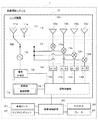•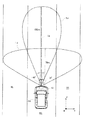•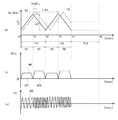•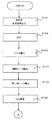•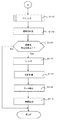•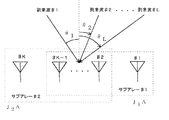•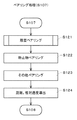•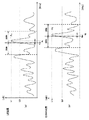•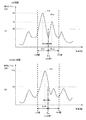•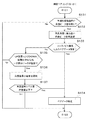•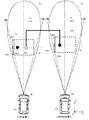•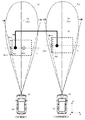•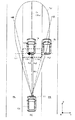•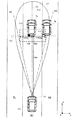•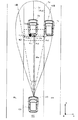•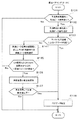•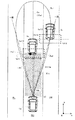•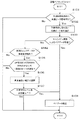•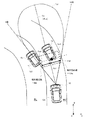•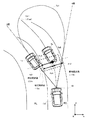•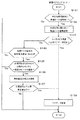••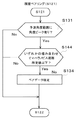••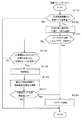## Classifications

• GPHYSICS
• G01MEASURING; TESTING
• G01S13/00Systems using the reflection or reradiation of radio waves, e.g. radar systems; Analogous systems using reflection or reradiation of waves whose nature or wavelength is irrelevant or unspecified
• G01S13/02Systems using reflection of radio waves, e.g. primary radar systems; Analogous systems
• G01S13/06Systems determining position data of a target
• BPERFORMING OPERATIONS; TRANSPORTING
• B60VEHICLES IN GENERAL
• B60WCONJOINT CONTROL OF VEHICLE SUB-UNITS OF DIFFERENT TYPE OR DIFFERENT FUNCTION; CONTROL SYSTEMS SPECIALLY ADAPTED FOR HYBRID VEHICLES; ROAD VEHICLE DRIVE CONTROL SYSTEMS FOR PURPOSES NOT RELATED TO THE CONTROL OF A PARTICULAR SUB-UNIT
• B60W30/00Purposes of road vehicle drive control systems not related to the control of a particular sub-unit, e.g. of systems using conjoint control of vehicle sub-units, or advanced driver assistance systems for ensuring comfort, stability and safety or drive control systems for propelling or retarding the vehicle
• GPHYSICS
• G01MEASURING; TESTING
• G01S13/00Systems using the reflection or reradiation of radio waves, e.g. radar systems; Analogous systems using reflection or reradiation of waves whose nature or wavelength is irrelevant or unspecified
• G01S13/02Systems using reflection of radio waves, e.g. primary radar systems; Analogous systems
• G01S13/06Systems determining position data of a target
• G01S13/08Systems for measuring distance only
• G01S13/32Systems for measuring distance only using transmission of continuous unmodulated waves, amplitude-, frequency- or phase-modulated waves
• G01S13/34Systems for measuring distance only using transmission of continuous unmodulated waves, amplitude-, frequency- or phase-modulated waves using transmission of frequency-modulated waves and the received signal, or a signal derived therefrom, being heterodyned with a locally-generated signal related to the contemporaneous transmitted signal to give a beat-frequency signal
• G01S13/345Systems for measuring distance only using transmission of continuous unmodulated waves, amplitude-, frequency- or phase-modulated waves using transmission of frequency-modulated waves and the received signal, or a signal derived therefrom, being heterodyned with a locally-generated signal related to the contemporaneous transmitted signal to give a beat-frequency signal using triangular modulation
• GPHYSICS
• G01MEASURING; TESTING
• G01S13/00Systems using the reflection or reradiation of radio waves, e.g. radar systems; Analogous systems using reflection or reradiation of waves whose nature or wavelength is irrelevant or unspecified
• G01S13/02Systems using reflection of radio waves, e.g. primary radar systems; Analogous systems
• G01S13/06Systems determining position data of a target
• G01S13/42Simultaneous measurement of distance and other co-ordinates
• GPHYSICS
• G01MEASURING; TESTING
• G01S13/00Systems using the reflection or reradiation of radio waves, e.g. radar systems; Analogous systems using reflection or reradiation of waves whose nature or wavelength is irrelevant or unspecified
• G01S13/02Systems using reflection of radio waves, e.g. primary radar systems; Analogous systems
• G01S13/50Systems of measurement based on relative movement of target
• G01S13/58Velocity or trajectory determination systems; Sense-of-movement determination systems
• G01S13/583Velocity or trajectory determination systems; Sense-of-movement determination systems using transmission of continuous unmodulated waves, amplitude-, frequency-, or phase-modulated waves and based upon the Doppler effect resulting from movement of targets
• G01S13/584Velocity or trajectory determination systems; Sense-of-movement determination systems using transmission of continuous unmodulated waves, amplitude-, frequency-, or phase-modulated waves and based upon the Doppler effect resulting from movement of targets adapted for simultaneous range and velocity measurements
• GPHYSICS
• G01MEASURING; TESTING
• G01S13/00Systems using the reflection or reradiation of radio waves, e.g. radar systems; Analogous systems using reflection or reradiation of waves whose nature or wavelength is irrelevant or unspecified
• G01S13/93Radar or analogous systems specially adapted for specific applications for anti-collision purposes
• G01S13/931Radar or analogous systems specially adapted for specific applications for anti-collision purposes of land vehicles
• GPHYSICS
• G01MEASURING; TESTING
• G01S13/00Systems using the reflection or reradiation of radio waves, e.g. radar systems; Analogous systems using reflection or reradiation of waves whose nature or wavelength is irrelevant or unspecified
• G01S13/93Radar or analogous systems specially adapted for specific applications for anti-collision purposes
• G01S13/931Radar or analogous systems specially adapted for specific applications for anti-collision purposes of land vehicles
• G01S2013/932Radar or analogous systems specially adapted for specific applications for anti-collision purposes of land vehicles using own vehicle data, e.g. ground speed, steering wheel direction
• GPHYSICS
• G01MEASURING; TESTING
• G01S13/00Systems using the reflection or reradiation of radio waves, e.g. radar systems; Analogous systems using reflection or reradiation of waves whose nature or wavelength is irrelevant or unspecified
• G01S13/93Radar or analogous systems specially adapted for specific applications for anti-collision purposes
• G01S13/931Radar or analogous systems specially adapted for specific applications for anti-collision purposes of land vehicles
• G01S2013/9327Sensor installation details
• G01S2013/93271Sensor installation details in the front of the vehicles

## Description

The present invention relates to signal processing for deriving a position of a target.

2. Description of the Related Art Conventionally, a radar device provided in a vehicle outputs a transmission wave from a transmission antenna, receives a reflected wave from a target that reflects the transmission wave with a reception antenna, and positions of the target with respect to the vehicle (radar device). The target information including is derived. Specifically, the radar apparatus generates a beat signal by mixing a transmission signal corresponding to a transmission wave that is frequency-modulated at a predetermined period and a reception signal corresponding to a reflected wave.

Next, the radar apparatus performs FFT (Fast Fourier Transform) processing on the beat signal to generate a frequency spectrum, and extracts a signal (hereinafter referred to as “frequency peak signal”) exceeding a predetermined threshold in the frequency spectrum. Then, the radar apparatus performs pairing by combining the frequency peak signal in the UP section and the frequency peak signal in the DOWN section based on a predetermined condition, and determines the pair data. The radar apparatus derives data (hereinafter referred to as “filter data”) obtained by filtering the pair data of the current process and the data related to the target of the past process corresponding thereto.

Then, the radar apparatus derives a signal (hereinafter referred to as “predicted peak signal”) in which each frequency peak signal in the UP section and DOWN section of the current process is predicted based on the filter data of the previous process. This prediction peak signal has parameters such as a prediction frequency and a prediction angle. That is, the predicted peak signal is a signal indicating the predicted position of the target to be processed this time. The radar apparatus extracts the frequency peak signal (hereinafter referred to as “history peak signal”) of the current processing having temporal continuity with the filter data of the past processing in each of the UP section and the DOWN section based on the predicted frequency. To do.

Next, the radar apparatus performs an azimuth calculation based on the history peak signal, and then, in the angle spectrum derived by the azimuth calculation process, a peak signal (hereinafter referred to as an “angle peak signal”) that exceeds a predetermined threshold is referred to as an UP section and a peak value. Derived in each section of the DOWN section. The angle of the angle peak signal that is the target data corresponds to the angle of the target. Note that the maximum number of angle peak signals that can be derived from one frequency peak signal in the azimuth calculation is, for example, three.

The radar apparatus pairs the angle peak signal in the UP section and the angle peak signal in the DOWN section based on the predicted angle. Specifically, the radar apparatus combines an angle peak signal having an angle closest to the predicted angle in the UP section and an angle peak signal having an angle closest to the predicted angle in the DOWN section, and the signal level of the angle peak signal in both sections. If the difference is less than or equal to a predetermined value, the angle peak signal is based on the reflected wave from the same reflection point, and is determined as pair data (hereinafter referred to as “history pair data”) for the current process.

The radar apparatus derives the distance from the vehicle to the target, the angle of the target with respect to the vehicle, and the relative velocity of the target with respect to the vehicle based on the filter data obtained by filtering the history pair data. Information such as the position and relative speed of the target is output to the vehicle control device. As a result, the vehicle control device performs necessary vehicle control according to the position of the target. As a technique related to the present invention, there is, for example, Patent Document 1.

JP 2012-220196 A

However, even if the radar apparatus combines the angle peak signal of the UP section having the angle closest to the predicted angle and the angle peak signal of the DOWN section, the signal level difference between the angle peak signals of both sections exceeds a predetermined value. The angle peak signals in both sections are not determined as pair data.

Here, the reason why the signal level difference between the angle peak signals in both sections exceeds a predetermined value is that the angle peak signal in the UP section and the angle peak signal in the DOWN section are peak signals from different reflection points. The reason why the angle peak signals at different reflection points are close to the predicted angle is as follows. There is a time difference of about several milliseconds between the timing at which the radar device receives the reflected wave corresponding to the angle peak signal in the UP section and the timing at which the reflected wave corresponding to the angle peak signal in the DOWN section is received. This is because the location where the transmission wave in the UP section is reflected and the location where the transmission wave in the DOWN section is reflected are different due to the movement of the host vehicle or the target during the period. In addition, a reflected wave from a vehicle traveling in front of the host vehicle (hereinafter referred to as “front vehicle”) within the own lane in which the host vehicle travels can obtain a relatively large number of reflection points. This is because if the reflection point is limited to the maximum number of angle peak signals that can be derived in the azimuth calculation, a minute error may occur between the angle of the angle peak signal and the original angle of the target.

Thus, when the signal level difference between the angle peak signal in the UP section to be combined and the angle peak signal in the DOWN section exceeds a predetermined value, the radar apparatus cannot derive the history pair data in the current process. As a result, the radar apparatus assumes that data obtained by predicting the history pair data of the current process from the filter data of the previous process is the history pair data of the current process (hereinafter referred to as “extrapolation process”). ). When such extrapolation processing is performed, a position different from the actual position of the target may be derived, which may reduce the reliability of the target information.

The present invention has been made in view of the above problems, and an object of the present invention is to provide a technique for determining a correct combination as pair data while minimizing extrapolation processing.

In order to solve the above-mentioned problem, the invention of claim 1 is a radar device that is provided in a vehicle and receives a reflected wave from a target and derives the position of the target, and performs transmission with frequency modulation at a predetermined period. Deriving means for deriving target data in each of the UP section in which the frequency of the signal increases and the DOWN section in which the frequency decreases, and the target data in the past processing in each of the UP section and the DOWN section. Setting means for setting a prediction area which is a range of a predicted position of the target data of the current process based on the target data of the current process in the prediction area of at least one of the UP section and the DOWN section there determination means for determining whether there exist a plurality of the first angle peak signal which is the target object data of a position closest to the predicted position of the UP section of the DOWN section The combination of the first angle peak signal and the second angle peak signal when the combination with the second angle peak signal that is the target data closest to the predicted position satisfies the pairing condition, Pairing means for confirming as pair data, and the pairing means, when the combination of the first angle peak signal and the second angle peak signal does not satisfy the pairing condition, There are a plurality of angle peak signals that are the target data in the prediction area of the section, and a combination of angle peak signals other than the combination of the first angle peak signal and the second angle peak signal is When a re-pairing condition that is different from the pairing condition is satisfied, a combination of the other angle peak signals is combined with the pair data. To confirm Te.

According to a second aspect of the present invention, in the radar device according to the first aspect, the pairing means has a Mahalanobis distance based on a combination of the first angle peak signal and the second angle peak signal equal to or less than a predetermined value. In this case, it is determined that the pairing condition is satisfied, and an angle difference between two angle peak signals from among other combinations of angle peak signals other than the combination of the first angle peak signal and the second angle peak signal Is selected, and it is determined that the re-pairing condition is satisfied when the signal level difference between the two angle peak signals having the smallest angle difference is a predetermined value or less.

Further, the invention of claim 3, in the radar device according to claim 1 or 2, wherein the pairing means, as the vertical distance of the target object data is increased, the product narrows the angle range of the prediction region The combination of the target data is determined as pair data.

According to a fourth aspect of the present invention, in the radar device according to any one of the first to third aspects, an acquisition means for acquiring a lane route on which the vehicle travels, and a relative lateral distance of the target data is calculated based on the lane route. calculating means for, further wherein the pairing means, to determine a combination of the target object data in the own traffic lane based on the relative transverse distance as the pair data.

The invention of claim 5 is the radar apparatus according to claim 1, setting means for setting a plurality of regions based on the angle at the predicted region, further wherein the pairing means, the plurality of regions The combination of the target data existing in the combinable region is determined as pair data.

A sixth aspect of the invention includes the radar apparatus according to any of the first to fifth aspects, and a vehicle control apparatus that controls the behavior of the vehicle based on target information from the radar apparatus.

The invention according to claim 7 is a signal processing method for deriving the position of the target by receiving a reflected wave from the target and provided in the vehicle, and (a) a transmission signal that is frequency-modulated at a predetermined period. A step of deriving target data in each of the UP section in which the frequency increases and the DOWN section in which the frequency decreases, and (b) the target data in the past processing in each of the UP section and the DOWN section. A step of setting a prediction region that is a range of a predicted position of the target data of the current processing based on the step, (c) within the prediction region of at least one of the UP section and the DOWN section, a first angle peak signal target data is the target object data position closest whether there exist a plurality of the predicted position of the determining step and; (d) UP period, the said DOWN section When the combination of the second angle peak signal that is the target data closest to the measurement position satisfies the pairing condition, the combination of the first angle peak signal and the second angle peak signal is determined as the pair. And the step (d) includes at least one section when a combination of the first angle peak signal and the second angle peak signal does not satisfy the pairing condition. A plurality of angle peak signals, which are the target data, exist in the prediction region, and a combination of angle peak signals other than the combination of the first angle peak signal and the second angle peak signal is the pair. When the re-pairing condition that is different from the ring condition is satisfied, the combination of the other angle peak signals is determined as the pair data.

According to the first to seventh aspects of the invention, even when there are a plurality of target data in the prediction region, it becomes easy to determine the correct combination as pair data, the extrapolation processing can be reduced, and the object actually exists. The position to perform can be derived. In addition, according to the invention of claim 1 in particular, the correct combination can be determined as pair data in both cases where the combination of the target data at the position closest to the predicted position satisfies the predetermined condition and when the combination does not satisfy the predetermined condition, The position where the object actually exists can be derived.

In particular, according to the invention of claim 3, the target data existing outside the own lane in which the vehicle is traveling can be excluded from the selection target of the re-pairing process as data existing outside the prediction region, It is possible to prevent the determined combination from being determined as pair data.

In particular, according to the invention of claim 4 , even when the vehicle travels in a curve lane, only target data existing in the own lane on which the vehicle travels can be used as a pairing candidate. Can be prevented.

In particular, according to the invention of claim 5 , in the UP section and the DOWN section, it is possible to eliminate the combination of the target data existing in the unpairable area, and to prevent the wrong combination from being determined as the pair data. .

FIG. 1 is a block diagram of a vehicle control system FIG. 2 is a diagram illustrating a transmission range of a transmission wave of the transmission antenna. FIG. 3 is a diagram illustrating a beat signal derived based on a transmission signal and a reception signal. FIG. 4 is a flowchart of target information derivation processing performed by the signal processing unit. FIG. 5 is a flowchart of target information derivation processing performed by the signal processing unit. FIG. 6 is a diagram for explaining the processing contents of ESPRIT. FIG. 7 is a flowchart for explaining history pairing, stationary object pairing, and other pairing processing. FIG. 8 is a diagram for explaining history peak extraction. FIG. 9 is a diagram for explaining derivation of an angle peak signal within a predicted angle range and selection of an angle peak signal having an angle closest to the predicted angle. FIG. 10 is a flowchart for explaining history pairing processing. FIG. 11 is a diagram illustrating a combination of angle peak signals having an angle closest to the predicted angle. FIG. 12 is a diagram showing a combination in which the angle difference is the smallest and the angle signal level difference is a predetermined value or less. FIG. 13 is a diagram for explaining a prediction region in a case where the angle peak signal exists at a relatively short distance. FIG. 14 is a diagram for explaining a prediction region when the angle peak signal exists at a relatively long distance. FIG. 15 is a diagram illustrating a state in which the angle range is narrowed as the vertical distance of the angle peak signal increases. FIG. 16 is a flowchart of the process according to the second embodiment. FIG. 17 is a diagram illustrating the own lane region in the region where the angle peak signal to be repaired exists. FIG. 18 is a flowchart of the process according to the third embodiment. FIG. 19 is a diagram illustrating a state in which an angle peak signal of an adjacent vehicle is included in a prediction area in a curve lane. FIG. 20 is a diagram illustrating selection of a re-pairing process candidate based on the relative lateral distance. FIG. 21 is a flowchart of the process according to the fourth embodiment. FIG. 22 is a diagram illustrating a combination candidate of the angle peak signal in the prediction area of the UP section and the angle peak signal in the prediction area of the DOWN section. FIG. 23 is a flowchart of the process according to the fifth embodiment. FIG. 24 is a diagram illustrating a state in which the prediction area of the UP section and the DOWN section is divided into a plurality of areas. FIG. 25 is a flowchart of processing according to the sixth embodiment.

Hereinafter, embodiments of the present invention will be described with reference to the drawings.

<1. System block diagram>
<1-1. Overall configuration>
FIG. 1 is a block diagram of the vehicle control system 1. The vehicle control system 1 is a system that controls the behavior of the host vehicle, and includes a radar device 10, a vehicle control device 20, a vehicle speed sensor 21, a steering sensor 22, a throttle 23, and a brake 24. The radar device 10 and the vehicle control device 20 are electrically connected. The vehicle control device 20 is electrically connected to a vehicle speed sensor 21, a steering sensor 22, a throttle 23, and a brake 24.

The vehicle control device 20 performs vehicle control based on the target information acquired from the radar device 10. For example, the vehicle control device 20 controls ACC (Adaptive Cruise Control) that travels following the preceding vehicle while securing a predetermined inter-vehicle distance between the host vehicle and the preceding vehicle based on the target information of the preceding vehicle.

The vehicle speed sensor 21 outputs a signal corresponding to the speed of the host vehicle to the vehicle control device 20 based on the rotation speed of the axle of the host vehicle. The vehicle control device 20 derives the current speed of the host vehicle based on a signal from the vehicle speed sensor 21.

The steering sensor 22 derives the rotation angle of the steering wheel by the operation of the driver of the host vehicle and outputs it to the vehicle control device 20. The vehicle control device 20 derives the value of the curve radius related to the route of the own lane on which the host vehicle travels based on the information acquired from the steering sensor 22 and outputs the value to the radar device 10.

The throttle 23 increases the speed of the host vehicle by the operation of the driver of the host vehicle. The throttle 23 increases the speed of the host vehicle under the control of the vehicle control device 20. For example, the throttle 23 increases the speed of the host vehicle so that the distance between the host vehicle and the preceding vehicle is maintained at a constant value in the ACC control.

The brake 24 reduces the speed of the host vehicle by the operation of the driver of the host vehicle. The brake 24 reduces the speed of the host vehicle under the control of the vehicle control device 20. For example, the brake 24 reduces the speed of the host vehicle so that the distance between the host vehicle and the preceding vehicle is maintained at a constant value in the ACC control.

Next, the radar apparatus 10 will be described. The radar apparatus 10 includes an antenna 101, a mixer 13 (13a to 13d), an AD (Analog to Digital) converter 14 (14a to 14d), a signal generation unit 15, an oscillator 16, a switch SW, and a storage unit. 17 and a signal processing unit 18.

The antenna 101 includes a transmission antenna 11 and a reception antenna 12. The transmission antenna 11 includes two antennas, transmission antennas 11a and 11b. The transmission antenna 11 is switched at a predetermined cycle by switching of the switch SW. As a result, at least one of the two transmission antennas outputs a transmission wave.

The reception antenna 12 includes four antennas, that is, reception antennas 12a, 12b, 12c, and 12d. The four receiving antennas receive the reflected wave from the target and output the received signal to the mixer 13.

<1-2. Transmission range>
The transmission ranges of the transmission antennas 11a and 11b will be described with reference to FIG. FIG. 2 is a diagram illustrating a transmission range of transmission waves of the transmission antennas 11a and 11b. In FIG. 2, directions are described using xyz coordinate axes. The xyz coordinate axes are fixed relative to the host vehicle CA (hereinafter referred to as “vehicle CA”). The vehicle width direction of the vehicle CA corresponds to the x-axis direction, and the traveling direction of the vehicle CA corresponds to the y-axis direction. Further, the height direction (vehicle height direction) of the vehicle CA corresponds to the z-axis direction. FIG. 2 is a view of the vehicle CA as viewed from above (+ z side) from above (+ z side) in the height direction (z-axis direction).

The transmission wave from the transmission antenna 11a is output to the range of the transmission range Tr1. The horizontal beam direction of the transmission range Tr1 is the extending direction of the transmission axis Ce of the radar apparatus 10, and is the same direction as the traveling direction of the vehicle CA. The transmission range Tr1 is a range of ± about 7 degrees, that is, about 14 degrees with respect to the transmission axis Ce when the transmission axis Ce is ± 0 degrees in the horizontal angle range. The transmission distance of this transmission wave is set to about 150 m. The horizontal angle range of the transmission range Tr1 is a range including the width (about 3.6 m) of the own lane RC when the vehicle CA is located at the approximate center of the own lane RC.

Further, the transmission wave from the transmission antenna 11b is output in the range of the transmission range Tr2. The horizontal beam direction of the transmission range Tr2 is the extending direction of the transmission axis Ce, and is the same direction as the traveling direction in which the vehicle CA moves forward. The transmission range Tr2 is a range of ± about 30 degrees, that is, about 60 degrees with respect to the transmission axis Ce in the horizontal angle range. The transmission distance of this transmission wave is set to about 70 m. The horizontal angle range of the transmission range Tr2 is that when the vehicle CA is located substantially in the center of the own lane RC, the own lane RC, the left lane RL adjacent to the left side of the own lane RC, and the right side adjacent to the right side of the own lane RC. This is a range that includes the width of the lane RR (approximately 3.6 m x 3 = approximately 10.8 m).

Note that the transmission wave output from the transmission antenna 11b is used to correctly derive the direction of the target even if phase wrapping occurs when performing the calculation of the direction of the target described later. The phase folding means that the phase of the reflected wave from the target rotates 360 degrees to derive an angle different from the original angle at which the target exists. The radar apparatus 10 outputs transmission waves in different transmission ranges (transmission ranges Tr1 and Tr2) from the transmission antenna 11a and the transmission antenna 11b, and an accurate angle of the target according to the reception level of the reflected wave corresponding to each transmission wave Is derived.

Returning to FIG. 1, the mixer 13 has four mixers 13 a to 13 d. The mixer 13 is provided in each receiving antenna (12a to 12d). The mixer 13 mixes the reception signal corresponding to the reflected wave received by the reception antenna 12 and the transmission signal corresponding to the transmission wave. The mixer 13 outputs a beat signal, which is a difference signal between the reception signal and the transmission signal, to the AD converter 14.

The AD converter 14 has four AD converters, AD converters 14a, 14b, 14c, and 14d. The AD converter 14 is provided in each of the receiving antennas 12a, 12b, 12c, and 12d. The AD converter 14 converts the analog signal input from the mixer 13 into a digital signal. Specifically, the AD converter 14 samples a beat signal that is an analog signal at a predetermined period. The AD converter 14 quantizes the sampled beat signal, converts it into a digital signal, and outputs it to the signal processing unit 18.

The signal generation unit 15 generates a modulation triangular wave signal and outputs it to the oscillator 16. The oscillator 16 frequency-modulates a triangular wave signal with a millimeter wave band (for example, 76.5 GHz) signal using the triangular wave signal, and outputs the result to the switch SW.

The switch SW is connected to one of the transmission antennas 11a and 11b. The switch SW switches the transmission antenna to be connected every predetermined timing (for example, 5 msec). The switch SW outputs a transmission signal that is a millimeter-wave band signal to one of the transmission antennas 11a and 11b.

<1-3. Explanation of each signal>
Next, each signal of the transmission signal, the reception signal, and the beat signal will be described with reference to the drawings. FIG. 3 is a diagram for explaining the beat signal BS derived based on the transmission signal TS and the reception signal RS. In addition, each symbol used in FIG. 3 and the numerical formula demonstrated below represents the following content. f 0 : center frequency of transmission wave, ΔF: frequency deviation width, f m : repetition frequency of modulation wave, c: speed of light (radio wave speed), T: round-trip time of radio wave between vehicle CA and target, f s : transmission / reception frequency, R: longitudinal distance, V: relative speed.

FIG. 3A shows signal waveforms of the transmission signal TS and the reception signal RS. FIG. 3B is a diagram showing the beat frequency generated by the difference between the transmission signal TS and the reception signal RS. FIG. 3C shows a beat signal BS corresponding to the beat frequency.

In FIG. 3A, the vertical axis represents frequency [GHz] and the horizontal axis represents time [msec]. The transmission signal TS in the figure has a frequency f 0 (for example, 76.5 GHz) as a center frequency, rises to a predetermined frequency (for example, 76.6 GHz), and then decreases to a predetermined frequency (for example, 76.4 GHz) for 200 MHz. Repeat a certain change.

The transmission signal TS has a section in which the frequency rises to a predetermined frequency (hereinafter referred to as “UP section”). The UP section corresponds to the section U1 (time t0 to t1) and the section U2 (time t2 to t3). The transmission signal TS has a section (hereinafter referred to as “DOWN section”) in which the transmission signal TS rises to a predetermined frequency and then falls to a predetermined frequency. The DOWN section corresponds to the section D1 (time t1 to t2) and the section D2 (time t3 to t4). The first transmission section Tx1 (time t0 to t2) is a section in which the transmission antenna 11a outputs a transmission wave. The second transmission section Tx2 (time t2 to t4) is a section in which the transmission antenna 11b outputs a transmission wave.

The signal processing section Tx3 (time t4 to t5) is a section in which the signal processing unit 18 performs a process of deriving the position of the target based on the transmission signal TS and the reception signal RS. Note that the reception signal RS has an UP section in which the frequency increases to a predetermined frequency and a DOWN section in which the frequency decreases to the predetermined frequency, as in the case of the transmission signal TS.

A time delay (time T) occurs between the transmission signal TS and the reception signal RS according to the distance of the target with respect to the vehicle CA. When there is a speed difference between the speed of the vehicle CA and the speed of the target, a difference corresponding to the Doppler shift occurs in the reception signal RS with respect to the transmission signal TS.

In FIG. 3B, the vertical axis represents frequency [kHz] and the horizontal axis represents time [msec]. The beat frequency BF in the figure is the frequency of the difference between the transmission signal TS and the reception signal RS in the UP section and the DOWN section. For example, the beat frequency BF1 is derived in the section U1, and the beat frequency BF2 is derived in the section D1. Thus, in each section, the beat frequency BF indicating the frequency difference between the transmission signal TS and the reception signal RS is derived.

In FIG. 3C, the vertical axis represents amplitude [V], and the horizontal axis represents time [msec]. The beat signal BS in the figure is an analog signal corresponding to the beat frequency BF. The beat signal BS is converted from an analog signal to a digital signal by the AD converter 14. In addition, when the receiving antenna 12 receives a plurality of reflected waves from a plurality of reflection points of the target, beat signals corresponding to the plurality of reflected waves are derived.

Returning to FIG. 1, the storage unit 17 stores an execution program of various arithmetic processes executed by the signal processing unit 18. The storage unit 17 records a plurality of target information derived by the signal processing unit 18. For example, the storage unit 17 stores the target information 17a derived in the past process and the current process. The target information 17a is information including target position information and speed information. The position information includes the distance (hereinafter referred to as “vertical distance”) until the reflected wave reflected from the target is received by the receiving antenna 12, and the vehicle lateral direction (vehicle width direction) from the target position to the transmission axis Ce. ) Distance (hereinafter referred to as “lateral distance”). This lateral distance is derived by performing a trigonometric function operation on the target angle. The speed information is information including the relative speed of the target with respect to the vehicle CA.

The signal processing unit 18 derives target information based on the above-described beat signal BS derived from the transmission signal TS and the reception signal RS. The target information derivation process performed by the signal processing unit 18 will be described below with reference to the process flowcharts of FIGS. 4 and 5.

<2. Processing flowchart>
<2-1. Overall processing>
4 and 5 are flowcharts of the target information derivation process performed by the signal processing unit 18. The signal processing unit 18 outputs an instruction signal for generating a transmission wave to the signal generation unit 15 (step S101). The signal generation unit 15 generates a transmission wave corresponding to the transmission signal TS based on the instruction signal from the signal processing unit 18. The generated transmission wave is output outside the vehicle CA.

The receiving antenna 12 receives a reflected wave obtained by reflecting a transmission wave on a target, and outputs a received signal RS to the mixer 13. The reception signal RS and the transmission signal TS are mixed by the mixer 13, and a beat signal that is a difference signal between the transmission signal TS and the reception signal RS is generated. The beat signal BS is converted from an analog signal to a digital signal by the AD converter 14 and output to the signal processing unit 18.

The signal processing unit 18 performs FFT processing on the beat signal BS of the digital signal in each of the UP section and the DOWN section (step S102). As a result, the signal processing unit 18 acquires FFT data having a signal level value and phase information for each frequency related to the beat signal BS in each of the UP section and the DOWN section. The FFT data is acquired for each of the receiving antennas 12a to 12d.

The signal processing unit 18 performs a “peak extraction” process of extracting a signal whose signal level value exceeds a predetermined threshold from the frequency spectrum in the FFT data as a frequency peak signal (step S103). In this peak extraction process, frequency peak signals are extracted in each of the UP section and the DOWN section, and the number of frequency peak signals is determined.

Then, the signal processing unit 18 extracts the frequency peak signal of the target of the current process that is temporally continuous with the target derived by the past process from the frequency peak signal extracted by the peak extraction process. The processing of “peak extraction” is performed (step S104). Detailed processing contents of the history peak extraction will be described later.

Next, the signal processing unit 18 determines that the frequency difference between the frequency peak signal in the UP section and the frequency peak signal in the DOWN section corresponds to the own vehicle speed of the vehicle CA from the information on the own vehicle speed of the vehicle speed sensor 40 of the vehicle CA, and the signal level value is A “stationary object peak extraction” process is performed in which substantially the same frequency peak signal is extracted as a frequency peak signal corresponding to a stationary object (hereinafter referred to as “stationary object peak signal”) (step S105). Here, the stationary object is a target having a relative speed substantially the same as the speed of the vehicle CA. A target that moves at a specific speed and has a relative speed different from the speed of the vehicle CA is a moving object.

The signal processing unit 18 performs azimuth calculation based on the frequency peak signal using a predetermined azimuth calculation algorithm in each of the UP and DOWN sections (step S106). The azimuth calculation algorithm is, for example, ESPRIT (Estimation of Signal Parameters via Rotational Invariance Techniques). The signal processing unit 18 uses ESPRIT to derive an angle θ up corresponding to the angle peak signal in the UP section and an angle θ dn corresponding to the angle peak signal in the DOWN section. The signal processing unit 18 derives the angle θm of the target by the equation (1) by pairing the angle peak signal of the UP section and the angle peak signal of the DOWN section.

<2-2. Direction calculation by ESPRIT>
The ESPRIT process will be described. FIG. 6 is a diagram for explaining the processing contents of ESPRIT. ESPRIT is a technique of dividing the receiving antennas 12a to 12d into two subarrays whose positions are shifted, and estimating the arrival direction of the incoming wave (reflected wave) from the phase difference between the two subarrays.

In FIG. 6, the antenna is a K-element linear array, the number of incoming waves is L, and the angle of the i-th incoming wave is θ i (i = 1, 2,..., L). ESPRIT is a technique for estimating the phase rotation of each incoming wave caused by the parallel movement of the entire array based on the rotation invariance “J 1 AΦ = J 2 A”. The matrix J 1 and the matrix J 2 are (K−1) × K transformation matrices. K is the number of receiving antennas 12. A is a directional matrix composed of array response vectors each having θ 1 to θ L as variables. Φ is an L-order diagonal matrix.

In FIG. 6, in the linear array of K elements, the first to (K-1) th elements are subarrays # 1, and the second to Kth elements are subarrays # 2. Thus, J 1 A of the rotation invariant means that the 1st to (K−1) th rows of the matrix A are extracted and the J 2 A is the 2nd to Kth rows of the matrix. That is, J 1 A represents the direction matrix of subarray # 1. J 2 A represents the direction matrix of subarray # 2.

If A is known here, Φ is obtained and the arrival angle of the path is estimated. However, since A is to be estimated, Φ cannot be directly solved. Therefore, the K × K covariance matrix R xx of the K-dimensional received signal vector is obtained. And R xx is eigenvalue expansion using the eigenvector corresponding to the larger eigenvalue than the thermal noise power sigma 2 from the resulting eigenvalues, a signal subspace matrix E s is generated.

The generated signal subspace matrix E s and matrix A can be expressed as A = E s T −1 using an L-order regular matrix T that exists only between the two. Here E s is the K × L matrix. T is an L × L regular matrix. When the equation of A = E s T −1 is substituted into the rotation invariant, (J 1 E s ) (TΦT −1 ) = J 2 E s is obtained. If TΦT −1 is obtained from this equation and eigenvalue expansion is performed, the eigenvalue becomes a diagonal component of Φ. As a result, the angle of the incoming wave is estimated from the eigenvalue. Thereby, the signal processing unit 18 derives the same number of target angles as the number obtained by subtracting 1 from the total number of antennas of the receiving antenna 12 among a plurality of targets existing at different angles at a certain frequency. That is, the signal processing unit 18 can derive up to three angles of targets existing at substantially the same vertical distance using the four receiving antennas 12 of the radar apparatus 10.

Next, the processing flowchart of FIG. 5 will be described. The signal processing unit 18 performs pairing processing for pairing the peak signal in the UP section and the peak signal in the DOWN section (step S107). In this pairing process, pairing is performed by a different pairing method depending on the type of the frequency peak signal extracted.

Specifically, for the history peak signal extracted by the history peak extraction in step S104, the “history pairing” process is performed using the history peak signal in the UP section and the history peak signal in the DOWN section. For the stationary object peak signal extracted by the stationary object peak extraction in step S105, the “stationary object pairing” process is performed by the stationary object peak signal in the UP section and the stationary object peak signal in the DOWN section. Further, the remaining peak signals excluding the historical peak signal and the stationary object peak signal among all the peak signals extracted by the peak extraction in step S103 are the remaining peak signals in the UP section and the remaining peak signals in the DOWN section. The “other pairing” process is performed.

FIG. 7 is a flowchart for explaining history pairing, stationary object pairing, and other pairing processing. The signal processing unit 18 performs history pairing processing using the history peak signal extracted by history peak extraction (step S121). The history pairing process is performed using, for example, the Mahalanobis distance. Specifically, from the two parameter values “angle difference” and “angle signal level difference” between the angle peak signal that is the target data in the UP section and the angle peak signal that is the target data in the DOWN section, UP The probability of the combination of the angle peak signal of the section and the angle peak signal of the DOWN section is derived from the equation (2). In equation (2), RD represents the Mahalanobis distance, θdg represents an angle difference, and θpg represents an angle signal level difference.

When the Mahalanobis distance is a predetermined value (for example, 60) or less, the signal processing unit 18 determines the combination of the angle peak signals in the UP section and the DOWN section as pair data. In addition, when the Mahalanobis distance by a certain pattern combination exceeds a predetermined value, the signal processing unit 18 examines whether or not pairing by another pattern combination is possible. This process will be described later.

Next, the signal processing unit 18 performs a stationary object pairing process using the stationary object peak signal extracted by the stationary object peak extraction (step S122). Of the angle peak signals corresponding to the stationary object peak signal, the signal processing unit 18 has an angle range of ± about 6 degrees with respect to the angle of the angle peak signal of the UP section, that is, a DOWN section existing in an angle range of about 12 degrees. An angle peak signal with the smallest angle difference between the angle peak signals in both sections is derived from the angle peak signal. The signal processing unit 18 is an angle peak signal of the UP section existing in an angle range of about ± 6 degrees, that is, an angle range of about 12 degrees with reference to the angle peak signal angle of the DOWN section. An angle peak signal with the smallest angle difference is derived. And the signal processing unit 18 is a case where the combination of the smallest angle difference viewed from the UP section side and the combination of the smallest angle difference viewed from the DOWN section side is a combination of the same angle peak signals. The difference in signal level value is a predetermined value (for example,
When it is within 20 dB), this combination is determined as pair data.

Next, the signal processing unit 18 performs other pairing processing using the remaining peak signals excluding the historical peak signal and the stationary object peak signal among all peak signals extracted by the peak extraction processing (step S123). . The signal processing unit 18 performs other pairing processing using, for example, the Mahalanobis distance shown in Equation (2), and determines the pair data with the combination having the smallest Mahalanobis distance as the correct combination.

Then, the signal processing unit 18 uses the value of the parameter of the confirmed pair data and the expressions (3) and (4) in the pairing process of steps S121 to S123, and the vertical distance of the confirmed pair data, A lateral distance based on the relative speed and the angle derived from the equation (1) is derived (step S124). In equations (3) and (4), fup represents the frequency of the frequency peak signal in the UP section, and fdn represents the frequency of the frequency peak signal in the DOWN section.

Returning to the process of step S108 of FIG. 5, the signal processing unit 18 continues in time between the current pair data paired by the current target derivation process and the previous pair data paired by the previous process. A continuity determination is performed to determine whether or not a relationship exists (step S108). Here, the case where the previous pair data and the current pair data have a temporally continuous relationship (there is continuity) is as follows. The signal processing unit 18 generates predicted pair data in which the current pair data is predicted based on the previous pair data, and the vertical distance, the horizontal distance, and the difference in relative speed between the current pair data and the predicted pair data are predetermined values. If it is within the range, the previous pair data and the current pair data are continuous. Thereby, it is determined that the target derived by the current process and the target derived by the past process are the same target. Note that the signal processing unit 18 determines that the current pair data having the smallest difference from the predicted pair data is the previous pair data when a plurality of current pair data has a difference value such as a longitudinal distance within a predetermined value. It is determined that the data has continuity.

In addition, when the difference between the vertical distance, the horizontal distance, and the relative speed between the current pair data and the predicted pair data exceeds a predetermined value, the signal processing unit 18 determines the time between the current pair data and the previous pair data. It is determined that there is no continuous relationship (no continuity). The pair data determined to have no continuity in this way becomes new pair data derived for the first time in the current process.

Next, the signal processing unit 18 determines whether or not the continuity of the current pair data is equal to or greater than a predetermined number (for example, twice) (step S109). For example, the current continuity of the pair data is derived as new pair data in the previous processing (no continuity), and there is continuity with the pair data of the previous processing in the previous processing (first continuity). This is a case where the processing has continuity with the pair data of the previous processing (continuity second time). As described above, when the signal processing unit 18 determines that the same target has been continuously derived in time in the process of the previous time, the previous time, and the current time at least three times (Yes in step S109), this time Filter processing is performed on the pair data (step S110). Note that the signal processing unit 18 ends the process when the continuity of the pair data is less than twice this time (No in step S109).

Returning to the process of step S110, the signal processing unit 18 performs filtering of the values of the vertical distance, the relative speed, the horizontal distance, and the signal level between the current pair data and the predicted pair data (step S110). Filter data that is pair data is derived as target information of the target of the current process.

In the filtering process, for example, the signal processing unit 18 weights the lateral distance of the predicted pair data with a value of 0.75 for the lateral distance, weights the lateral distance of the current pair data with a value of 0.25, and adds both values together. Is obtained as the lateral distance of the filter data of this processing. Filter processing is similarly performed for the values of the vertical distance, the relative speed, and the signal level.

Next, the signal processing unit 18 derives a predicted peak signal (predicted frequency, predicted angle, etc.) used for the history peak extraction process in step S104 in the process (next process) performed next to the current process (step S111). ). Specifically, the signal processing unit 18 derives 20 target information items with high priority in performing vehicle control, and from the target information of this processing in each of the UP section and the DOWN section of the target information. The predicted frequency and predicted angle for the next process are calculated. Then, the signal processing unit 18 performs history peak extraction based on the predicted frequency as described later, and performs history pairing based on the predicted angle. Note that the predicted frequency of the UP section is different from the predicted frequency of the DOWN section in accordance with the relative speed of the target. Since the prediction angle is an angle of one target, the prediction angle in the UP section and the prediction angle in the DOWN section are the same angle.

An example of the priority order of the target information in the control of the ACC is a moving object having a relatively small vertical distance from the vehicle CA. A target corresponding to a preceding vehicle traveling on the own lane has a high priority, and the vehicle A target corresponding to a stationary object having a relatively long vertical distance from the CA has a lower priority.

Next, the signal processing unit 18 performs a data combination process for collecting a plurality of filter data into data corresponding to one object (step S112). For example, when the vertical distance and the horizontal distance of a plurality of filter data derived by the signal processing unit 18 are relatively short distances and the relative velocities are substantially the same, such a plurality of filter data is a plurality of reflections from one object. There is a high possibility that the data corresponds to a point. Therefore, the signal processing unit 18 combines a plurality of filter data into one and handles the same object data.

Then, the signal processing unit 18 outputs, to the vehicle control device 20, the filter data having a high priority for the necessity of vehicle control among the filter data obtained by data combination in the process of step S112 (step S113).

<3. History peak extraction and history pairing>
Next, the detailed contents of history peak extraction (step S104 in FIG. 4) and history pairing (step S121 in FIG. 7) will be specifically described with reference to FIGS.

FIG. 8 is a diagram for explaining history peak extraction. The vertical axis in FIG. 8 indicates the signal level [dB], and the horizontal axis indicates the frequency [kHz]. FIG. 8A shows history peak extraction in the UP section, and FIG. 8B shows history peak extraction in the DOWN section. In each of the UP section and the DOWN section, the signal processing unit 18 has a high frequency side and a low frequency side based on the prediction frequency derived by the next prediction in the previous process (step S111 in FIG. 5). Extracts the historical peak signal within the predicted frequency range of 3BIN. 1BIN is about 468Hz.

In the case shown in FIG. 8A, the frequency peak signal Pu (frequency fu, signal level value L1) is present in the predicted frequency range with the predicted frequency fu as a reference, and this frequency peak signal Pu is the predicted frequency fue. The peak signal with the frequency closest to. Note that the frequency peak signal Pu is a signal extracted in excess of the threshold signal level L0 in the peak extraction process of step S103. The signal processing unit 18 extracts a frequency peak signal Pu having a frequency closest to the predicted frequency fue within the predicted frequency range as the history peak signal Pu.

In the case shown in FIG. 8B, the frequency peak signal Pd (frequency fdn, signal level L1a) is present in the predicted frequency range with the predicted frequency fde as a reference, and this frequency peak signal Pd becomes the predicted frequency fde. The peak signal of the nearest frequency. Note that the frequency peak signal Pd is a signal extracted in excess of the threshold signal level L0 in the peak extraction process of step S103. The signal processing unit 18 extracts the frequency peak signal Pd having the frequency closest to the predicted frequency fde within the predicted frequency range as the history peak signal Pd.

Next, the signal processing unit 18 separates and derives the angle peak signal from the historical peak signal of the UP section and the DOWN section by the azimuth calculation, and outputs the angle peak signal within the predicted angle range in each of the UP section and the DOWN section. Deriving and selecting the angle peak signal of the angle closest to the predicted angle. FIG. 9 is a diagram for explaining derivation of an angle peak signal within a predicted angle range and selection of an angle peak signal having an angle closest to the predicted angle. In each of the UP section and the DOWN section, the signal processing unit 18 is an angle peak signal within an angle range of ± about 4 degrees with reference to the prediction angle derived by the next prediction of the previous process (step S111 in FIG. 5). And an angle peak signal having an angle closest to the predicted angle is selected.

In the case shown in FIG. 9A, the angle peak signals Pu1 and Pu2 exist within the predicted angle range based on the predicted angle θe (for example, ± 0 degrees), and the angle peak signal Pu2 is the predicted angle θe. Is the peak signal at the angle closest to. The angle peak signals Pu1 and Pu2 exceed the threshold signal level R0 for extracting the angle peak signal from the angle spectrum. In this way, the signal processing unit 18 derives the angle peak signals Pu1 and Pu2 within the predicted angle range, and selects the angle peak signal Pu2 as an angle peak signal having an angle closest to the predicted angle θe.

In the case shown in FIG. 9B, the angle peak signal Pd1 is present within the predicted angle range with the predicted angle θe as a reference, and the angle peak signal Pd1 is a peak signal having an angle closest to the predicted angle θe. The angle peak signal Pd1 is a signal that exceeds the threshold signal level R0 for extracting the angle peak signal from the angle spectrum. As described above, the signal processing unit 18 derives the angle peak signal Pd1 within the predicted angle range, and selects the angle peak signal Pd1 as the angle peak signal having the angle closest to the predicted angle θe.

As described above, conventionally, when the angle peak signals Pu2 and Pd1 closest to the predicted angle are selected, and the signal level difference of these angle peak signals satisfies the pair data determination condition, the pairing process is performed. If the condition is not satisfied, extrapolation processing is performed without determining the pair data. In the present embodiment, in contrast to such conventional processing, when the selected angle peak signals Pu2 and Pd1 do not satisfy the pair data determination condition, processing for not determining the pair data based on the combination of these angle peak signals is performed. The same processing is performed. However, before performing extrapolation processing, the signal processing unit 18 examines whether or not another combination satisfies a definite condition different from the above-described pair data definitive condition. That is, the signal processing unit 18 selects the angle peak signal Pu1 and Pd1 as another combination because the angle peak signal Pu1 different from the angle peak signal Pu2 exists in the predicted angle range of the UP section in the example of FIG. To do. Then, the signal processing unit 18 determines whether or not these combinations satisfy another definite condition. In other words, if the first selected combination of angle peak signals does not satisfy the pairing permission condition, it is determined whether or not the next selected combination of angle peak signals satisfies the re-pairing permission condition. . As described above, there are two types of pairing processes, “pairing” and “re-pairing”, in the history pairing process.

The history pairing process including the process of deriving the angle peak signal within the predicted angle range as described above and selecting the angle peak signal having the angle closest to the predicted angle θe will be described with reference to FIG. FIG. 10 is a flowchart for explaining history pairing processing. In FIG. 10, the signal processing unit 18 determines whether or not an angle peak signal exists in the predicted angle range (within ± about 4 degrees) with respect to the predicted angle θe in each of the UP section and the DOWN section. (Step S131). If there is no angle peak signal in the predicted angle range in either the UP section or the DOWN section (No in step S131), the signal processing unit 18 ends the process without determining the history pair data. If the history pair data is not determined, the signal processing unit 18 assumes that the history pair data of the current process is predicted from the filter data of the previous process as the history pair data of the current process in the continuity determination process in step S108. Perform extrapolation to temporarily secure the continuity of the target.

When there is an angle peak signal in the predicted angle range in each of the UP section and the DOWN section (Yes in step S131), the signal processing unit 18 has an angle peak signal having an angle closest to the predicted angle θe in each section. Is selected (step S132). Specifically, the signal processing unit 18 selects the angle peak signal Pu2 in the UP section described in FIG. 9 and the angle peak signal Pd1 in the DOWN section.

Next, the signal processing unit 18 determines whether or not the pairing combination using the Mahalanobis distance is confirmed as pair data, that is, whether or not the pairing permission condition is satisfied (step S133). For example, the signal processing unit 18 determines whether or not pair data has been determined by a combination as shown in FIG. FIG. 11 is a diagram illustrating a combination of angle peak signals having an angle closest to the predicted angle θe. The prediction area Su in the UP section has an intersection between the frequency fu of the frequency peak signal Pu and the prediction angle θe as a predicted position, and ± 3 BINs in the frequency direction (y-axis direction) from the predicted position, and the angular direction (x-axis direction) ) Is an approximately rectangular region corresponding to ± about 4 degrees. As described above, the prediction region is a region including a prediction frequency range and a prediction angle range, and is a range of a prediction position. The angle peak signal in the prediction area Su is a pairing combination candidate. Note that the shape of the prediction region may be a shape other than a rectangle (for example, a trapezoid or an ellipse).

In the example of FIG. 11, angle peak signals Pu1 and Pu2 exist in the prediction area Su. The signal processing unit 18 selects the angle peak signal Pu2 having an angle closest to the predicted angle θe as a combination target.

The prediction area Sd of the DOWN section is an area corresponding to ± about 3 BIN in the frequency direction from the prediction position and ± about 4 degrees in the angle direction with the intersection of the frequency fd of the frequency peak signal Pd and the prediction angle θe as the prediction position. It becomes. The angle peak signal in the prediction region Sd is a candidate for the history pairing combination. In the example of FIG. 11, the angle peak signal Pd1 exists in the region of the prediction region Sd. The signal processing unit 18 selects the angle peak signal Pd1 having an angle closest to the predicted angle θe as a combination target.

Returning to step S133 of FIG. 10, the signal processing unit 18 determines the probability of the combination of the angle peak signals using the Mahalanobis distance. Specifically, the signal processing unit 18 uses the above equation (2) to calculate the angle difference (θu2−θd1) and the angle signal level difference between the angle peak signal Pu2 in the UP section and the angle peak signal Pd1 in the DOWN section ( The Mahalanobis distance is derived from R2-R1a). When the Mahalanobis distance is equal to or smaller than the predetermined value (Yes in Step S133), the signal processing unit 18 determines the pairing of the angle peak signals Pu2 and Pu1 as pair data assuming that the pairing permission condition is satisfied (Step S134).

In the process of step S133, if the Mahalanobis distance exceeds a predetermined value (No in step S133), the signal processing unit 18 determines that the combination of the angle peak signals Pu2 and Pd1 is not a combination of the same target, and uses a combination based on the pattern. It is determined whether another combination satisfies the re-pairing permission condition without being confirmed as pair data.

Specifically, the signal processing unit 18 determines whether or not there are a plurality of angle peak signals in at least one of the UP section and the DOWN section (step S135), and the angle peak signal is at least in any section. When there are a plurality (Yes in Step S135), the combination having the smallest angle difference is selected from the combinations of different patterns (Step S136).

Then, the signal processing unit 18 determines whether or not the angle signal level difference between the angle peak signals having the smallest angle difference is equal to or less than a predetermined value (for example, 3.5 dB) (step S137), and the angle signal level difference is determined. If the value is equal to or smaller than the predetermined value (Yes in step S137), the combination is determined as pair data (step S134).

Specifically, the signal processing unit 18 determines a combination as shown in FIG. 12 as pair data. FIG. 12 is a diagram showing a combination in which the angle difference is the smallest and the angle signal level difference is a predetermined value or less. Two peak signals of the angle peak signals Pu1 and Pu2 exist in the region of the prediction region Su in the UP section, and the peak signal of the angle peak signal Pd1 exists in the region of the prediction region Sd in the DOWN section. In such a case, the condition that there are a plurality of angle peak signals in at least one of the sections of step S135 in FIG. 10 described above is satisfied. Then, the signal processing unit 18 excludes the angular difference signal except the angular peak signal Pu2 used for determining whether or not the pairing permission condition is satisfied among the angular peak signals in the prediction region Su and the prediction region Sd. The combination of the angle peak signals Pu1 and Pd1 that minimizes is derived as a target for determining whether or not the re-pairing permission condition is satisfied.

As described above, the signal processing unit 18 uses the angle peak signal of one section used to determine the pairing permission condition among the angle peaks of both sections used to determine whether or not the pairing permission condition is satisfied. In addition, the re-pairing permission condition is determined based on another angle peak signal that is not used for determining the pairing permission condition in the other section.

In the case shown in FIG. 12, since only one angle peak signal Pd1 exists in the prediction area Sd in the DOWN section, in the process of selecting the combination with the smallest angle difference in step S136, the angle peak signal Pd1 Is selected. However, when another angle peak signal different from the angle peak signal Pd1 exists in the prediction region Sd, the angle difference between the other angle peak signal and the angle peak signal Pu1 is larger than the angle peak signal Pd1. When the angle is smaller, another angle peak signal different from the angle peak signal Pd1 is selected.

When the angle signal level difference between the angle peak signals Pu1 and Pd1 is less than or equal to a predetermined value, the condition that the angle peak signal level difference in step S137 of FIG. 10 is less than or equal to the predetermined value is satisfied, and the signal processing unit 18 pairs this combination. Confirm as data. Thereby, when there are a plurality of angle peak signals in the prediction region, the correct combination can be determined as pair data while minimizing extrapolation processing, and the position where the object actually exists can be derived.

In addition, when there are not a plurality of angle peak signals in at least one of the UP section and the DOWN section in step S135 (No in step S135), or when the angle signal level difference between the angle peak signals in step S137 exceeds a predetermined value ( In step S137, No), the signal processing unit 18 ends the process without confirming the history pair data, and performs the extrapolation process in the process of step S108.

As described above, in the present embodiment, the signal processing unit 18 performs the combination of the angle peak signals at positions closest to the predicted position based on the predicted frequency and the predicted angle in each of the UP section and the DOWN section. When the combination of the angle peak signals satisfies the pairing permission condition using the Mahalanobis distance, the signal processing unit 18 determines the pair data as the most likely combination.

If the combination of the angle peak signals at the position closest to the predicted position does not satisfy the pairing permission condition using the Mahalanobis distance, the signal processing unit 18 satisfies the repairing condition that is another pairing. If the re-pairing condition is satisfied, the most probable combination is determined as pair data. As a result, the combination of the angle peak signal at the position closest to the planned position can be confirmed as the pair data regardless of whether the pairing condition is met or not, and the object can be detected while reducing extrapolation as much as possible. The actual location can be derived.

The permission condition for the re-pairing process is that there are a plurality of angle peak signals in at least one of the UP section and the DOWN section, and the angle difference between the angle peak signal in the UP section and the angle peak signal in the DOWN section. Is a condition as to whether or not the signal level difference of the smallest combination is equal to or less than a predetermined value.

<Second Embodiment>
Next, a second embodiment will be described. In the history pairing process described in the first embodiment, the signal processing unit 18 of the radar apparatus 10 according to the second embodiment narrows the angle range of the prediction region as the vertical distance of the target increases. Then, processing for determining the combination of angle peak signals as pair data is performed.

The predicted angle range, which is the angle range of the predicted region, becomes wider as the vertical distance of the target increases as the horizontal distance is changed even if the angle range remains constant (for example, ± 4 degrees). Therefore, when the target of the own lane exists at a relatively long distance, when the signal processing unit 18 performs the re-pairing process with another combination pattern as in the first embodiment, the adjacent lane or the like There is a possibility that an angle peak signal of a target existing outside the range of the own lane is included in the prediction region, and an incorrect combination is determined as pair data. The process described in the second embodiment is a process for preventing an incorrect combination from being determined as pair data.

The configuration and processing of the radar apparatus 10 of the second embodiment are substantially the same as those of the first embodiment, but the history pairing processing is partially different. Hereinafter, the differences will be mainly described with reference to FIGS. The angle peak signal is a signal obtained by angle-separating the frequency peak signal. Therefore, the frequency of the frequency peak signal corresponds to the vertical distance of the angle peak signal.

FIG. 13 is a diagram for explaining the prediction region Su1 when the angle peak signal is present at a relatively short distance from the vehicle CA. In the present embodiment, processing in the UP section is described as an example, but processing in the DOWN section is performed in the same manner as the processing in the UP section. The angle peak signals Pu1, Pu2 and Pu3 in the UP section are signals obtained by angle-separating the frequency peak signal of the frequency fu1. The frequency fu1 corresponds to a vertical distance of about 30 m, for example. The prediction area Su1 is an area of ± about 3 BIN in the frequency direction (y-axis direction) and ± about 4 degrees in the angle direction (x-axis direction) from the predicted position of the intersection of the frequency fu1 and the prediction angle θe. The lateral distance of the prediction area Su1 is in the range of about ± 1.8 m, that is, about 3.6 m when the predicted angle θe is the lateral distance ± 0 m, and includes the own lane RC. Therefore, only the angle peak signals Pu1 and Pu2 of the forward vehicle Ta traveling in the own lane RC are included in the prediction area Su1. In other words, the angle peak signal Pu3 of the adjacent vehicle Tb traveling in the right lane RR is not included in the region of the prediction region Su1.

Next, a case where the angle peak signal exists at a relatively long distance from the vehicle CA will be described. FIG. 14 is a diagram for explaining the prediction region Su2 when the angle peak signal exists at a relatively long distance. In FIG. 14, the angle peak signals Pu1, Pu2, and Pu3 in the UP section are signals obtained by angle-separating the frequency peak signal of the frequency fu2 corresponding to the longitudinal distance of about 60 m. The prediction area Su2 is an area of ± about 3 BIN in the frequency direction (y-axis direction) and ± about 4 degrees in the angle direction (x-axis direction) from the predicted position of the intersection of the frequency fu2 and the prediction angle θe. The lateral distance of the prediction area Su2 is within the range of lateral distance ± about 4.2 m, that is, about 8.4 m when the prediction angle θe is the lateral distance ± 0 m, and includes the own lane RC and the right lane RR and the left lane RL of the adjacent lane. It becomes a range. Therefore, the prediction area Su2 includes the angle peak signals Pu1 and Pu2 of the front vehicle Ta traveling in the own lane RC and the angle peak signal Pu3 of the adjacent vehicle Tb traveling in the right lane RR. As described above, since the angle peak signals of the preceding vehicle Ta and the adjacent vehicle Tb are subjected to the re-pairing process, when the signal processing unit 18 performs the re-pairing process with another combination pattern, an error occurs in the process. There is a possibility that pair data will be determined by the combination.

Therefore, the signal processing unit 18 performs a process of narrowing the angle range of the prediction region as the vertical distance of the angle peak signal increases. FIG. 15 is a diagram illustrating a state in which the angle range is narrowed as the vertical distance of the angle peak signal increases. As shown in FIG. 15, the signal processing unit 18 uses the angle that is a candidate for the re-pairing process from within the prediction region Su3 that has an angle range of ± about 2 degrees that is narrower than the prediction region Su1 that has an angle range of ± about 4 degrees. Select the peak signal. Specifically, the signal processing unit 18 selects only the angle peak signal Pu1 and Pu2 of the forward vehicle Ta traveling on the own lane RC in the prediction area Su3 as candidates for the re-pairing process combination. In other words, the signal processing unit 18 does not select the angle peak signal Pu3 of the adjacent vehicle Tb traveling in the right lane RR outside the prediction region Su3 as a combination candidate. Thereby, the radar apparatus 10 can exclude the angle peak signal existing outside the range of the own lane RC on which the vehicle CA travels in the re-pairing process from the candidates for the re-pairing process.

In addition, the value of the prediction angle range of the prediction region according to the vertical distance of the angle peak signal is an example, and if the angle range can exclude the angle peak signal existing outside the range of the own lane RC, There may be. For example, when the vertical distance of the angle peak signal is about 50 m, the angle range of the prediction area may be about ± 2 degrees, and when the vertical distance of the angle peak signal is 100 m, the angle range of the prediction area may be about ± 1 degree. .

FIG. 16 is a flowchart of the process according to the second embodiment. When the Mahalanobis distance exceeds a predetermined value (No in step S133), the signal processing unit 18 sets a predicted angle range according to the vertical distance of the angle peak signal, and selects an angle peak signal within the predicted angle range (step) S141). That is, the signal processing unit 18 obtains an angle peak signal within a predetermined angle range (for example, ± about 4 degrees, that is, an angle range of about 8 degrees) in each of the UP section and the DOWN section in the process of step S131. In step S141, an angle peak signal within the predicted angle range corresponding to the vertical distance of the angle peak signal is selected. For example, when the vertical distance of the angle peak signal is 30 m, the predicted angle range is ± about 4 degrees, so the same angle peak signal as the angle peak signal selected in step S131 is selected. When the vertical distance of the angle peak signal is 60 m, the predicted angle range is about ± 2 degrees, so an angle peak signal that exists within an angle range narrower than the predetermined angle range is selected.

The signal processing unit 18 selects an angle peak signal that exists within an angle range corresponding to the vertical distance of the frequency peak signal (step S141), and then performs a re-pairing process (step S135 to step S137) that searches for a combination. )I do. In this way, the radar apparatus 10 narrows the angle range of the prediction area as the vertical distance of the angle peak signal increases, so that the angle peak signal outside the range of the own lane RC on which the vehicle CA travels can be re-paired. Only angle peak signals existing in the range of the own lane RC can be used as candidates for re-pairing.

In the process of the second embodiment, the signal processing unit 18 selects an angle peak signal within a predetermined angle range in each of the UP section and the DOWN section in the process of step S131, and then performs the step. It has been described that the angle peak signal within the predicted angle range corresponding to the vertical distance of the angle peak signal is selected in the process of S141. As other processing, the signal processing unit 18 may first perform processing for selecting an angle peak signal within a predicted angle range corresponding to the longitudinal distance of the angle peak signal. That is, the signal processing unit 18 may perform the process of step S141 instead of the process of step S131.

<Third Embodiment>
Next, a third embodiment will be described. In the signal processing unit 18 of the radar apparatus 10 according to the third embodiment, the angle peak signal that is target data is relatively close to the vehicle CA in the history pairing process described in the first embodiment. In the case of target data existing at a distance and at a position in the own lane RC where the vehicle CA travels, re-pairing processing (step S135 to step S137 in FIG. 10) is performed. In other words, the signal processing unit 18 is present when the angle peak signal is present at a relatively far position with respect to the vehicle CA, or in the adjacent lane of at least one of the left lane RL and the right lane RR. When the vehicle does not exist at a position in the traveling own lane RC, the re-pairing process is not performed and only the pairing process based on the predicted angle is performed. By such processing, the signal processing unit 18 can reduce the processing load when deriving the target information, and a pair with an incorrect combination of the angle peak signal existing in the own lane and the angle peak signal existing in the adjacent lane. Data confirmation can be prevented.

The configuration and processing of the radar apparatus 10 of the third embodiment are substantially the same as those of the first embodiment, but the history pairing processing is partially different. Hereinafter, the differences will be mainly described with reference to FIGS.

FIG. 17 is a diagram illustrating the own lane region ML in a region where the angle peak signal to be repaired exists. The own lane region ML is approximately 30 m from the vehicle CA when the vehicle CA is located approximately at the center of the own lane RC and the position is set to a vertical distance of 0 m, and the position of the vehicle CA is set to a lateral distance ± 0 m. This is a rectangular area with a lateral distance of about ± 1.5 m, that is, about 3.0 m. Note that the shape of the own lane region ML may be a shape other than a rectangle (for example, a trapezoid or an ellipse). In the present embodiment, processing in the UP section is described as an example, but processing in the DOWN section is performed in the same manner as the processing in the UP section.

When the position of the filter data that is the target of the past processing is within the own lane region ML, the signal processing unit 18 also automatically outputs the angle peak signal of the current processing that is a peak signal that is temporally continuous with this filter data. If the angle peak signal does not satisfy the pairing permission condition as being present in the lane region ML, the re-pairing process is performed. Specifically, the angle peak signals Pu1 and Pu2 of the forward vehicle Ta shown in FIG. 17 are present in the prediction region Su11 of the prediction frequency range based on the frequency fu1 and the prediction angle range based on the prediction angle θe. Each filter data corresponding to each of the angle peak signals Pu1 and Pu2 is present in the own lane region ML.

On the other hand, the angle peak signal Pu12 of the adjacent vehicle Tb exists in the prediction region Su12 of the prediction frequency range based on the frequency fu12 and the prediction angle range based on the prediction angle (for example, +4 degrees). The filter data of the angle peak signal Pu12 exists outside the own lane region ML in the previous process.

As described above, the signal processing unit 18 uses the angle peak signal Pu1 and the angle peak signal Pu2 in the area of the own lane area ML as the target of the pairing process based on the predicted angle, and satisfies the permission condition for pairing. If not, it will be the target of re-pairing processing.

Further, the signal processing unit 18 sets the angle peak signal Pu12 outside the area of the own lane area ML as a target for the pairing process based on the predicted angle, and when the filter data does not satisfy the permission condition for the pairing, the signal processing unit 18 Without extrapolation.

FIG. 18 is a flowchart of the process according to the third embodiment. When the Mahalanobis distance exceeds a predetermined value (No in step S133), the signal processing unit 18 determines whether or not the filter data of the previous process is within the own lane area ML (step S142). That is, it is determined whether or not the data for which continuity is to be determined is the highest priority data for vehicle control such as ACC control.

When the filter data of the previous process is within the own lane area ML (Yes in step S142), the signal processing unit 18 uses the angle peak signal of the current process corresponding to this filter data and the combination selected in step S132. Re-pairing processing (step S135 to step S137) is performed with an angle peak signal of a combination different from that for re-pairing processing. As described above, when the filter data of the past processing exists in the own lane region ML, the radar apparatus 10 performs pairing processing on the angle peak signal having temporal continuity with the filter data, If the ring permission conditions are not satisfied, the re-pairing process is performed. Further, when the filter data of the past process exists outside the own lane area ML, the radar apparatus 10 performs only the pairing process on the angle peak signal corresponding to the filter data, and the permission condition for pairing is satisfied. If not, extrapolation is performed without re-pairing. As a result, the radar apparatus 10 reduces the processing load when deriving the target information and determines the pair data with an incorrect combination of the angle peak signal existing in the own lane CR and the angle peak signal existing in the adjacent lane. Can be prevented.

<Fourth embodiment>
Next, a fourth embodiment will be described. The signal processing unit 18 of the radar apparatus 10 according to the fourth embodiment uses only the angle peak signal existing in the own lane range based on the relative lateral distance in the history pairing process described in the first embodiment. The target of re-pairing processing.

Specifically, the signal processing unit 18 performs the following processing. The signal processing unit 18 acquires the value of the curve radius related to the route of the own lane RC on which the vehicle CA travels from the vehicle control device 20. The value of the curve radius is derived by the vehicle control device 20 from the rotation angle by the steering sensor 22. The curve radius may be derived by other means such as road shape recognition by a camera. Then, the signal processing unit 18 calculates the relative lateral distance of the angle peak signal that is the target data based on the value of the curve radius, and the relative lateral distance is determined as the target of the re-pairing process. To do.

Here, the lateral distance includes an absolute lateral distance and a relative lateral distance. The absolute lateral distance is a distance in a direction substantially orthogonal to the transmission axis when the transmission axis Ce extending in the direction in which the transmission wave from the radar apparatus 10 is transmitted is defined as a lateral distance ± 0 m. The relative lateral distance refers to a distance in a direction substantially perpendicular to an axis (for example, the reference axis Ce1 in FIG. 20) obtained by bending the transmission axis Ce based on the value of the curve radius, in other words, a distance in a direction orthogonal to the own lane. When the lateral distance is derived, the signal processing unit 18 derives at least one of the two lateral distances for one target.

When the vehicle CA travels on a lane having a curve radius equal to or smaller than a predetermined value, if the signal processing unit 18 sets all the angle peak signals in the prediction region as targets for re-pairing, it is adjacent to the target. Sometimes it includes the angle peak signal of an adjacent vehicle traveling in the lane. As a result, an incorrect combination may be determined as pair data. In the fourth embodiment, when the vehicle CA travels on a lane having a curve radius equal to or smaller than a predetermined value, an angle peak signal that causes a wrong combination to be determined as pair data is re-paired as a candidate for a pairing combination. It is processing for excluding from.

The configuration and processing of the radar apparatus 10 of the fourth embodiment are substantially the same as those of the first embodiment, but the history pairing processing is partially different. Hereinafter, differences will be mainly described with reference to FIGS. In the present embodiment, processing in the UP section is described as an example, but processing in the DOWN section is performed in the same manner as the processing in the UP section.

In a curve lane where the curve radius is equal to or less than a predetermined value, the signal processing unit 18 is located in the region of the prediction area so that the signal processing unit 18 is in the predicted region by tilting the vehicle body in the steering rotation direction and the transmission axis Ce in the same direction as the vehicle body tilt. In some cases, an angle peak signal was included. FIG. 19 is a diagram illustrating a state in which the angle peak signal Pu4 of the adjacent vehicle Tc is included in the prediction area Su5 in the curve lane. As shown in FIG. 19, the signal processing unit 18 exists in the left lane RL of the vehicle body when the vehicle body of the vehicle CA is tilted with the rotation of the steering near the entrance of the curve and the transmission axis Ce is tilted in the same direction as the vehicle body. In some cases, the angle peak signal Pu4 of the adjacent vehicle Tc is included in the range of the prediction region Su5 and is used as a candidate for a combination of re-pairing processing.

Therefore, the signal processing unit 18 selects a re-pairing combination candidate based on the relative lateral distance condition together with the prediction area condition. The signal processing unit 18 acquires the value of the curve radius from the vehicle control device 20 and derives the relative lateral distance by the following equation (5). In the equation (5), Srd represents a relative lateral distance, Sad represents an absolute lateral distance, and CR represents a curve radius. The absolute lateral distance is a lateral distance derived using a trigonometric function from the angle of the pair data derived from the above equation (1). Therefore, the relative lateral distance of the angle peak signal is the relative lateral distance of the filter data of the previous process corresponding to the angle peak signal of the current process.

FIG. 20 is a diagram illustrating selection of a re-pairing process candidate based on the relative lateral distance. Of the angle peak signals in the prediction area Su5, the signal processing unit 18 has a relative lateral distance of about 1.8 m when the reference axis Ce1 is ± 0 m, that is, an angle peak signal Pu1 existing in a range of about 3.6 m. Only Pu2 and Pu2 are candidates for a re-pairing combination. In other words, the signal processing unit 18 combines the angle peak signal Pu4 outside the range of the relative lateral distance −1.8 m (for example, the relative lateral distance −2.2 m) with the re-pairing process even within the prediction area Su5. Not a candidate for Thus, even when the vehicle CA travels in a curved lane, the radar apparatus 10 can use only the angle peak signal existing in the own lane RC on which the vehicle CA travels as a candidate for the re-pairing process.

FIG. 21 is a flowchart of the process according to the fourth embodiment. When the Mahalanobis distance exceeds the predetermined value (No in step S133), the signal processing unit 18 uses a different combination different from the combination selected in step S132 in each of the UP section and the DOWN section. It is determined whether or not the relative lateral distance of the angle peak existing in the region is within about ± 1.8 m (step S143). When the relative lateral distance of the angular peak signal of another combination existing in the prediction area of the UP section and the DOWN section is within about ± 1.8 m (Yes in step S143), the signal processing unit 18 performs pairing again. Ring processing (step S135 to step S137) is performed. The signal processing unit 18 ends the process when the relative lateral distance of the angle peak signal exceeds about ± 1.8 m (No in step S143) in at least one of the UP section and the DOWN section. Thereby, even when the vehicle CA travels in a curve lane, the radar apparatus 10 can make only the angle peak signal present in the own lane RC on which the vehicle travels as a candidate for re-pairing.

<Fifth embodiment>
Next, a fifth embodiment will be described. The signal processing unit 18 of the radar apparatus 10 according to the fifth embodiment uses the angle peak signal in the prediction area of the UP section and the prediction area of the DOWN section in the history pairing process described in the first embodiment. Pairing is performed with the most probable combination among all the combinations with the angle peak signal. The configuration and processing of the radar apparatus 10 according to the fifth embodiment are partly different from the history pairing processing according to the first embodiment. Hereinafter, the difference will be mainly described with reference to FIGS.

FIG. 22 is a diagram showing candidates for combinations of the angle peak signal in the prediction area Su6 in the UP section and the angle peak signal in the prediction area Sd6 in the DOWN section. The angle peak signals Pu1, Pu2, and Pu3 in the region of the prediction area Su6 in the UP section are angle peak signals that are angle-separated from the frequency peak signal of the frequency fu3. The angle peak signals Pd1 and Pd3 in the prediction area Sd6 in the DOWN section are angle peak signals that are angle-separated from the frequency peak signal of the frequency fd3. The signal processing unit 18 performs a pairing process using the Mahalanobis distance based on all combinations of the angle peak signals Pu1, Pu2, and Pu3 and the angle peak signals Pd1 and Pd3. In this case, the signal processing unit 18 determines whether the six combinations satisfy the pairing permission condition.

FIG. 23 is a flowchart of the process according to the fifth embodiment. The signal processing unit 18 determines whether or not an angle peak signal exists within the predicted angle range (within ± about 4 degrees) with the predicted angle θe as a reference in both the UP section and the DOWN section (step S131). ). If there is no angle peak signal in the predicted angle range in either the UP section or the DOWN section (No in step S131), the signal processing unit 18 ends the process without determining the pair data.

When there is an angle peak signal within the predicted angle range (Yes in step S131), the signal processing unit 18 has a Mahalanobis distance that is equal to or less than a predetermined value (for example, 60 or less) among all combinations of the angle peak signals in the UP section and the DOWN section. ) Is determined whether or not there is a combination (step S144). When there is a combination in which the Mahalanobis distance is equal to or smaller than the predetermined value (Yes in Step S144), the signal processing unit 18 determines the combination as pair data (Step S134). In addition, the signal processing part 18 complete | finishes a process, when the combination whose Mahalanobis distance becomes below a predetermined value does not exist (No in step S144). In addition, when there are a plurality of combinations in which the Mahalanobis distance is a predetermined value or less, the signal processing unit 18 determines the combination having the smallest Mahalanobis distance as pair data. As a result, the radar apparatus 10 can reliably determine the pair data in the correct combination even when there are a plurality of angle peak signals in the prediction area, and can derive the position where the object actually exists.

<Sixth Embodiment>
Next, a sixth embodiment will be described. In the history pairing process described in the first embodiment, the signal processing unit 18 of the radar apparatus 10 according to the sixth embodiment sets a plurality of areas in the prediction area and performs the re-pairing process. At this time, an area that can be re-paired among a plurality of areas is selected based on the position of the angle peak signal in the prediction area. The configuration and processing of the radar apparatus 10 of the sixth embodiment are partly different from the history pairing processing of the first embodiment. Hereinafter, the difference will be mainly described with reference to FIGS. 24 and 25.

FIG. 24 is a diagram illustrating a state in which the prediction area of the UP section and the DOWN section is divided into a plurality of areas. The signal processing b18 sets three areas of the center area TE, the left area LE, and the right area RE based on the angles in the prediction area Su of the UP section and the prediction area Sd of the DOWN section. The center region TE, the left region LE, and the right region RE are set as ranges having different frequencies within the same range (± about 3 BIN) in the prediction region Su (Sd). Specifically, the central area TE is in the range of angle -2 degrees to +2 degrees, the left area LE is in the range of angle -2 degrees to -4 degrees,
The right region RE is set as a range of angle +2 degrees to +4 degrees.

These areas are set when the signal processing unit 18 performs the re-pairing process. When an angle peak signal is present in the center area TE of one section, the angle peak signal and the center area of the other section are set. The angle peak signal existing in any one of TE, the left region LE, and the right region RE can be combined. When an angle peak signal is present in the left region LE of one section, the angle peak signal is combined with the angle peak signal present in one of the left region LE and the center region TE of the other section. And is not combined with the angle peak signal present in the right region RE of the other section.

Further, when an angle peak signal is present in the right region RE of one section, a combination of the angle peak signal and an angle peak signal present in one of the right region RE and the center region TE of the other section And the angle peak signal present in the left region LE of the other section cannot be combined. That is, when the angle peak signal is present in the left or right region of one section, the signal processing unit 18 does not combine the angle peak signal with the angle peak signal present in the opposite region of the other section. .

Specifically, as shown in FIG. 24, the signal processing unit 18 calculates an angle peak signal Pu2 closest to the predicted angle (θe) of the UP section and an angle peak signal Pd1 closest to the predicted angle (θe) of the DOWN section. In combination, when the Mahalanobis distance exceeds a predetermined value, the prediction area Su (Sd) is divided into three areas.

The signal processing unit 18 sets another combination pattern different from the combination of the angle peak signal Pu2 and Pd1. That is, the signal processing unit 18 selects a combination of angle peak signals in the DOWN section based on the left region LE where the angle peak signal Pu1 exists. For the angle peak signal Pu1, the signal processing unit 18 selects the angle peak signal Pd1 existing in the left region LE of the DOWN section as a combination candidate. Then, when the angle signal level difference of the combination of the angle peak signals Pu1 and Pd1 is equal to or less than a predetermined value, the signal processing unit 18 determines the combination of the two as pair data.

If another angle peak signal is present in the center area TE of the DOWN section, the other angle peak signal is also a target of combination with the angle peak signal Pu1, and the signal processing unit 18 is connected to the angle peak signal Pu1. A combination with an angle peak signal having a smaller angle signal level difference is determined as pair data. If another angle peak signal is present in the right area RE of the DOWN section, the signal processing unit 18 does not make this other angle peak signal a candidate for a combination with the angle peak signal Pu1. As described above, the radar apparatus 10 excludes a combination of angle peak signals having greatly different angles from the pairing target, such as an angle peak signal existing in the left region LE and an angle peak existing in the right region RE. , Pair data by correct combination can be determined.

FIG. 25 is a flowchart of processing according to the sixth embodiment. When the Mahalanobis distance exceeds a predetermined value (No in step S133), the signal processing unit 18 determines whether or not there are a plurality of angle peak signals in at least one of the UP section and the DOWN section in the re-pairing process. Determination is made (step S135). When there are a plurality of angle peak signals in at least one of the sections (Yes in step S135), the signal processing unit 18 determines a plurality of areas (a center area TE, a left area LE, and a left area LE based on the angle of the prediction area Su (Sd)). , Right region RE) is set (step S145).

Then, the signal processing unit 18 selects the combination of the angle peak signals in the UP section and the DOWN section that have the smallest angle difference between the angle peak signals among the angle peak signals that exist in the combinable region (step S146). . When the angle signal level difference is equal to or smaller than the predetermined value (Yes in Step S137), the signal processing unit 18 determines the pair data by the combination (Step S134). Thereby, the radar apparatus 10 can exclude the combination of the angle peak signals existing in the uncombinable area, and can determine the correct combination existing in the combinable area as the pair data.

Note that the number of the plurality of regions in the prediction region is an example, and a number other than three (for example, four, etc.) may be set, and the angle value for dividing the region is also shown in the embodiment. The value may be different from the angle value.

<4. Modification>
Although the embodiments of the present invention have been described above, the present invention is not limited to the above-described embodiments, and various modifications are possible. Below, such a modification is demonstrated. All the forms including the above-described embodiment and the form described below can be appropriately combined.

In each of the above embodiments, the number of transmitting antennas 11 and receiving antennas 12 has been described as two and four, respectively, but the number of transmitting antennas 11 and the number of receiving antennas 12 may be other than the above. Good.

In each of the above embodiments, the transmission ranges Tr1 and Tr2 have been described with specific values indicating the angle at which the transmission axis Ce is ± 0 deg. However, these angles are examples, and other angles are used. There may be.

In each of the above embodiments, the vertical distance of the target has been described as the distance until the reflected wave reflected from the target is received by the receiving antenna 12 of the radar apparatus 10. On the other hand, the vertical distance of the target is the distance until the target is received by the receiving antenna 12 and the angle of the target when the target angle exists at an angular position other than the transmission axis Ce (± 0 deg). It is good also as a distance when it is assumed by calculation of the used trigonometric function that the angle of the target is on the transmission axis Ce (± 0 deg).

Further, in each of the above embodiments, the angle estimation method used by the radar apparatus 10 has been described by taking ESPRIT as an example. However, any one of angle estimation methods such as DBF (Digital Beam Forming), PRISM (Propagator method based on an Improved Spatial-smoothing Matrix), and MUSIC (Multiple Signal Classification) may be used.

Moreover, in each said embodiment, the radar apparatus 10 may be used for apparatuses other than vehicle CA. For example, the radar apparatus 10 may be used for an aircraft, a ship, and the like.

Further, in each of the above embodiments, it has been described that various functions are realized in software by the arithmetic processing of the CPU according to the program. However, some of these functions are realized by an electrical hardware circuit. Also good. Conversely, some of the functions realized by the hardware circuit may be realized by software.

DESCRIPTION OF SYMBOLS 1 Radar apparatus 10 Vehicle control system 11 Transmission antenna 12 Reception antenna 13 Mixer 14 AD conversion part 15 Signal generation part 16 Oscillator 17 Storage part 18 Signal processing part

## Claims (7)

1. A radar device provided in a vehicle for receiving a reflected wave from a target and deriving a position of the target,
Deriving means for deriving target data in each of an UP section in which the frequency of a transmission signal frequency-modulated at a predetermined period increases and a DOWN section in which the frequency decreases;
Setting means for setting a prediction area that is a range of a predicted position of the target data of the current process based on the target data of the past process in each of the UP section and the DOWN section;
Determining means for determining whether or not there are a plurality of the target data of the current process in the prediction region of at least one of the UP section and the DOWN section;
A first angle peak signal that is the target data at a position closest to the predicted position in the UP section, and a second angle peak signal that is the target data at a position closest to the predicted position in the DOWN section. Pairing means for determining a combination of the first angle peak signal and the second angle peak signal as the pair data when the combination satisfies a pairing condition;
With
When the combination of the first angle peak signal and the second angle peak signal does not satisfy the pairing condition, the pairing means uses the target data in the prediction area of the at least one section. Re-pairing in which a plurality of angle peak signals exist and a combination of other angle peak signals other than the combination of the first angle peak signal and the second angle peak signal is different from the pairing condition When satisfying the condition of, to determine the combination of the other angle peak signal as the pair data,
A radar device characterized by the above.
2. The radar apparatus according to claim 1, wherein
The pairing means determines that the pairing condition is satisfied when a Mahalanobis distance based on a combination of the first angle peak signal and the second angle peak signal is a predetermined value or less, and the first angle peak signal And the second angle peak signal other than the combination of the angle peak signals, the combination that minimizes the angle difference between the two angle peak signals is selected, and the angle difference is the minimum combination. Determining that the re-pairing condition is satisfied when a signal level difference between the two angle peak signals is equal to or less than a predetermined value;
A radar device characterized by the above.
3. The radar apparatus according to claim 1 or 2,
The pairing means determines the combination of the target data as pair data by narrowing the angle range of the prediction area as the vertical distance of the target data increases,
A radar device characterized by the above.
4. The radar apparatus according to any one of claims 1 to 3 ,
Obtaining means for obtaining a lane route on which the vehicle travels;
A calculation means for calculating a relative lateral distance of the target data based on the lane route;
Further comprising
The pairing means determines the combination of the target data in the own lane as pair data based on the relative lateral distance ;
A radar device characterized by the above.
5. The radar apparatus according to claim 1, wherein
Setting means for setting a plurality of regions based on angles within the prediction region;
Further comprising
The pairing means determines the combination of the target data existing in a combinable area among the plurality of areas as pair data ,
A radar device characterized by the above.
6. A radar device according to any one of claims 1 to 5;
A vehicle control device for controlling the behavior of the vehicle based on the target information from the radar device;
A vehicle control system comprising:
7. A signal processing method provided in a vehicle for receiving a reflected wave from a target and deriving a position of the target,
(A) Deriving target data in each of an UP section in which the frequency of a transmission signal frequency-modulated at a predetermined period increases and a DOWN section in which the frequency decreases;
(B) in each of the UP section and the DOWN section, setting a prediction region that is a range of a predicted position of the target data of the current process based on the target data of the past process;
(C) determining whether or not there are a plurality of the target data of the current process in the prediction region of at least one of the UP section and the DOWN section;
(D) a first angle peak signal that is the target data at a position closest to the predicted position in the UP section, and a second angle peak that is the target data at a position closest to the predicted position in the DOWN section. A combination of the first angle peak signal and the second angle peak signal is determined as the pair data when a combination with a signal satisfies a pairing condition;
With
In the step (d), when the combination of the first angle peak signal and the second angle peak signal does not satisfy the pairing condition, the target data is included in the prediction region of the at least one section. A pair of angle peak signals other than the combination of the first angle peak signal and the second angle peak signal is in a condition different from the pairing condition. Determining a combination of the other angle peak signals as the pair data when a ring condition is satisfied,
A signal processing method characterized by the above.
JP2014009227A 2014-01-22 2014-01-22 Radar apparatus, vehicle control system, and signal processing method Active JP6313981B2 (en)

## Priority Applications (1)

Application Number Priority Date Filing Date Title
JP2014009227A JP6313981B2 (en) 2014-01-22 2014-01-22 Radar apparatus, vehicle control system, and signal processing method

## Applications Claiming Priority (3)

Application Number Priority Date Filing Date Title
JP2014009227A JP6313981B2 (en) 2014-01-22 2014-01-22 Radar apparatus, vehicle control system, and signal processing method
DE102014118065.8A DE102014118065A1 (en) 2014-01-22 2014-12-08 radar
US14/564,599 US9869761B2 (en) 2014-01-22 2014-12-09 Radar apparatus

## Publications (2)

Publication Number Publication Date
JP2015137915A JP2015137915A (en) 2015-07-30
JP6313981B2 true JP6313981B2 (en) 2018-04-18

# Family

## Family Applications (1)

Application Number Title Priority Date Filing Date
JP2014009227A Active JP6313981B2 (en) 2014-01-22 2014-01-22 Radar apparatus, vehicle control system, and signal processing method

## Country Status (3)

US (1) US9869761B2 (en)
JP (1) JP6313981B2 (en)
DE (1) DE102014118065A1 (en)

## Families Citing this family (21)

* Cited by examiner, † Cited by third party
Publication number Priority date Publication date Assignee Title
KR20200084913A (en) 2014-02-27 2020-07-13 텔레폰악티에볼라겟엘엠에릭슨(펍) Method and apparatus for pyramid vector quantization indexing and de-indexing of audio/video sample vectors
JP2016151425A (en) * 2015-02-16 2016-08-22 パナソニックＩｐマネジメント株式会社 Radar system
US10598781B2 (en) * 2015-05-29 2020-03-24 Maxlinear, Inc. Cooperative and crowd-sourced multifunctional automotive radar
US9846228B2 (en) 2016-04-07 2017-12-19 Uhnder, Inc. Software defined automotive radar systems
US10261179B2 (en) * 2016-04-07 2019-04-16 Uhnder, Inc. Software defined automotive radar
WO2017175190A1 (en) 2016-04-07 2017-10-12 Uhnder, Inc. Adaptive transmission and interference cancellation for mimo radar
US9945935B2 (en) 2016-04-25 2018-04-17 Uhnder, Inc. Digital frequency modulated continuous wave radar using handcrafted constant envelope modulation
US9791551B1 (en) 2016-04-25 2017-10-17 Uhnder, Inc. Vehicular radar system with self-interference cancellation
US10573959B2 (en) 2016-04-25 2020-02-25 Uhnder, Inc. Vehicle radar system using shaped antenna patterns
WO2017187278A1 (en) 2016-04-25 2017-11-02 Uhnder, Inc. Pmcw – pmcw interference mitigation
US9954955B2 (en) 2016-04-25 2018-04-24 Uhnder, Inc. Vehicle radar system with a shared radar and communication system
WO2017187299A2 (en) 2016-04-25 2017-11-02 Uhnder, Inc. Successive signal interference mitigation
US9791564B1 (en) 2016-04-25 2017-10-17 Uhnder, Inc. Adaptive filtering for FMCW interference mitigation in PMCW radar systems
DE102016109910B4 (en) * 2016-05-30 2017-12-07 Infineon Technologies Ag Radar systems and method for operating a radar system
JP2017223589A (en) 2016-06-16 2017-12-21 株式会社デンソーテン Rader system and signal processing method
US9753121B1 (en) 2016-06-20 2017-09-05 Uhnder, Inc. Power control for improved near-far performance of radar systems
JP2017227511A (en) 2016-06-21 2017-12-28 株式会社デンソーテン Rader system and signal processing method
WO2018051288A1 (en) 2016-09-16 2018-03-22 Uhnder, Inc. Virtual radar configuration for 2d array
WO2018146632A1 (en) 2017-02-10 2018-08-16 Uhnder, Inc. Radar data buffering
KR101979818B1 (en) * 2017-07-31 2019-05-17 주식회사 에스원 Radar apparatus and control method thereof
US10534071B2 (en) * 2017-10-24 2020-01-14 Robert Bosch Gmbh Using data from a radar sensor for machine learning based perception

## Family Cites Families (32)

* Cited by examiner, † Cited by third party
Publication number Priority date Publication date Assignee Title
US5268692A (en) * 1991-03-14 1993-12-07 Grosch Theodore O Safe stopping distance detector, antenna and method
JPH06160518A (en) * 1992-11-18 1994-06-07 Mitsubishi Electric Corp On-vehicle radar device
JP3550829B2 (en) 1995-01-24 2004-08-04 株式会社デンソー FM-CW radar device
JPH09222477A (en) * 1996-02-19 1997-08-26 Toyota Motor Corp On-board radar apparatus
JP3383819B2 (en) * 1999-06-29 2003-03-10 株式会社ホンダエレシス FM-CW radar device
EP1369705B1 (en) * 2001-03-15 2009-12-30 Fujitsu Ten Limited Signal processing method for scanning radar
US6690319B2 (en) * 2001-11-08 2004-02-10 Fujitsu Ten Limited Scan type radar device
US6661370B2 (en) * 2001-12-11 2003-12-09 Fujitsu Ten Limited Radar data processing apparatus and data processing method
JP3753071B2 (en) * 2002-01-07 2006-03-08 株式会社村田製作所 Radar
JP3801068B2 (en) * 2002-02-19 2006-07-26 株式会社デンソー FMCW radar device, program
JP2003270341A (en) * 2002-03-19 2003-09-25 Denso Corp Signal processing device for on-vehicle radar and program
JP3975883B2 (en) * 2002-10-25 2007-09-12 株式会社デンソー Distance prediction method and radar apparatus
JP4016826B2 (en) * 2002-12-10 2007-12-05 株式会社デンソー Object labeling method and apparatus, program
JP2004205279A (en) * 2002-12-24 2004-07-22 Denso Corp Radar device and program
JP4093109B2 (en) * 2003-05-15 2008-06-04 株式会社デンソー Radar equipment for vehicles
JP4353184B2 (en) * 2004-01-07 2009-10-28 株式会社村田製作所 Radar
JP4287403B2 (en) * 2005-04-15 2009-07-01 本田技研工業株式会社 Object detection device
JP4919681B2 (en) * 2006-03-22 2012-04-18 Ｕｄトラックス株式会社 Automotive radar equipment
JP2008145178A (en) * 2006-12-07 2008-06-26 Denso Corp Adjusting method, direction detector, and electronic apparatus
JP4356758B2 (en) * 2007-03-20 2009-11-04 株式会社デンソー FMCW radar
JP2010096589A (en) * 2008-10-15 2010-04-30 Fujitsu Ten Ltd Signal processing device, radar device, and signal processing method
JP4905512B2 (en) * 2009-07-09 2012-03-28 株式会社デンソー Target information estimation device
JP5647814B2 (en) * 2010-05-19 2015-01-07 日本電産エレシス株式会社 Electronic scanning radar apparatus, received wave direction estimation method, and received wave direction estimation program
JP5491981B2 (en) * 2010-06-17 2014-05-14 株式会社東芝 Radar equipment
JP5709476B2 (en) * 2010-11-10 2015-04-30 富士通テン株式会社 Radar equipment
JP2012202955A (en) * 2011-03-28 2012-10-22 Fujitsu Ten Ltd Holographic radar
JP5628732B2 (en) 2011-04-04 2014-11-19 富士通テン株式会社 Arithmetic apparatus for radar apparatus, radar apparatus, arithmetic method and program for radar apparatus
JP5382087B2 (en) * 2011-11-02 2014-01-08 株式会社デンソー Radar equipment
JP6009788B2 (en) * 2012-03-21 2016-10-19 富士通テン株式会社 Radar apparatus and signal processing method
JP6004694B2 (en) * 2012-03-26 2016-10-12 富士通テン株式会社 Radar apparatus and target detection method
JP6077226B2 (en) * 2012-05-31 2017-02-08 富士通テン株式会社 Radar apparatus and signal processing method
JP6127554B2 (en) * 2013-02-08 2017-05-17 株式会社デンソー Radar equipment

## Also Published As

Publication number Publication date
US20150204971A1 (en) 2015-07-23
US9869761B2 (en) 2018-01-16
JP2015137915A (en) 2015-07-30
DE102014118065A1 (en) 2015-07-23

## Similar Documents

Publication Publication Date Title
US9958541B2 (en) Radar device, vehicle, and moving object speed detection method
JP3371854B2 (en) Ambient situation detection device and recording medium
US6249243B1 (en) Radar apparatus for use on vehicle
US8054216B2 (en) Radar device and target angle detection method
US7652616B2 (en) Target object detection system
JP6200644B2 (en) Radar apparatus and signal processing method
JP4093109B2 (en) Radar equipment for vehicles
KR100886437B1 (en) Fm-cw radar processing device
JP5062225B2 (en) Target detection device
JP2768439B2 (en) FM-CW type multi-beam radar device
DE19942665B4 (en) FM CW radar apparatus for measuring the distance to a target and the relative velocity of the target
JP4678945B2 (en) Scanning radar stationary object detection method
JP5320792B2 (en) Arrival direction estimation apparatus, arrival direction estimation method, and arrival direction estimation program
JP6548376B2 (en) Radar system, radar signal processing device, vehicle travel control device and method, and computer program
US10365350B2 (en) Neural network-based radar system having independent multibeam antenna
US7391361B2 (en) Signal processing method for FM-CW radar
JP4843003B2 (en) Signal processing apparatus, radar apparatus, and signal processing method
JP6534808B2 (en) Radar apparatus and signal processing method

## Legal Events

Date Code Title Description
A621 Written request for application examination

Free format text: JAPANESE INTERMEDIATE CODE: A621

Effective date: 20161125

A977 Report on retrieval

Free format text: JAPANESE INTERMEDIATE CODE: A971007

Effective date: 20170908

A131 Notification of reasons for refusal

Free format text: JAPANESE INTERMEDIATE CODE: A131

Effective date: 20170926

A521 Written amendment

Free format text: JAPANESE INTERMEDIATE CODE: A523

Effective date: 20171031

TRDD Decision of grant or rejection written
A01 Written decision to grant a patent or to grant a registration (utility model)

Free format text: JAPANESE INTERMEDIATE CODE: A01

Effective date: 20180314

A61 First payment of annual fees (during grant procedure)

Free format text: JAPANESE INTERMEDIATE CODE: A61

Effective date: 20180326

R150 Certificate of patent or registration of utility model

Ref document number: 6313981

Country of ref document: JP

Free format text: JAPANESE INTERMEDIATE CODE: R150